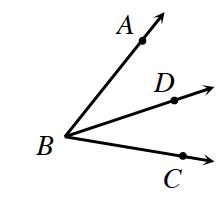### Home > INT2 > Chapter 6 > Lesson 6.2.2 > Problem6-66

6-66.

In the diagram below, $\overrightarrow { B D }$ bisects $∠ABC$. If $m∠ABD = 3x + 24°$ and $m∠CBD = 5x + 2°$, solve for $x$. Then determine $m∠ABC$

Using the definition of an angle bisector we know the two angles are congruent and the two expressions are equal.